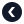Python专题Python 运算符

2021-07-02

1. 什么是运算符？
2. 算术运算符
3. 比较运算符
4. 逻辑运算符
5. 位运算符
6. 赋值运算符
7. 特殊运算符
8. 成员运算符

什么是运算符？

Python的运算符是一些用来进行算术运算或者逻辑运算的特殊符号。和运算符一起参与运算的值被称为操作数

3 + 5

算术运算符

+加法运算符对两个操作数进行加法运算3 + 8
+正号运算符表示一个数是正数+7
-减法运算符对两个操作数进行减法运算12 - 8
-负号运算符表示一个数是负数-5
*乘法运算符对两个操作数进行乘法运算3 * 6
/除法运算符对两个操作数进行除法运算8 / 4
%取余运算符计算两个操作数相除后的余数10 % 3
//地板除运算符(向下取整除)计算两个操作数相除的向下取整值10 // 3
**取余运算符计算两个操作数相除后的余数2 ** 3

比较运算符

>大于号运算符左侧值大于右侧值为True否则False8 > 5
<小于号运算符左侧值小于右侧值为True否则False8 < 5
==等于号运算符左侧值等于右侧值为True否则False8 == 5
!=不等号运算符左侧值不等于右侧值为True否则False8 != 5
>=大于或等于号运算符左侧值大于或等于右侧值为True否则False8 >= 5
<=小于或等于号运算符左侧值小于或等于右侧值为True否则False8 <= 5

逻辑运算符

and只有所有条件都为True，结果才为True，否则结果为False
or只要有一个条件为True时，结果就为True，否则结果为False
not当条件为True时，not TrueFalse，否则为True

1. b的值改为 True，看看结果如何变化。
2. ab的值都改为 False，再看看结果如何变化。

位运算符

&位与操作数两侧对应二进制位都为1时，此二进制位为1，否则为0

~位非操作数对应二进制位为1时，此二进制位为0，否则为1
^位异或操作数两侧对应二进制位不同是，此二进制位为1，否则为0
>>按位右移将操作数的每一位的往右移动n次，高位用符号位的值补齐
<<按位左移将操作数的每一位的往左移动n次，低位用0补齐

赋值运算符

x = 15

x = x + 20

# 等价于 x = x + 20
x += 20

=x = 2x = 2
+=x += 2x = x + 2
-=x -= 2x = x - 2
*=x *= 2x = x * 2
/=x /= 2x = x / 2
%=x %= 2x = x % 2
//=x //= 2x = x / 2
**=x **= 2x = x ** 2
&=x &= 2x = x & 2
|=x |= 2x = x | 2
^=x ^= 2x = x ^ 2
>>=x >>= 2x = x >> 2
<<=x <<= 2x = x << 2

特殊操作符

1. 标识运算符
2. 成员运算符

标识运算符

is如果操作数是指向同一块内存（指向相同的对象）则返回Truex is y
is not如果操作数不指向同一块内存（指向相同的对象）则返回Truex is not y

• 上面的xy是两个数组，在Python中创建的两个数组是两个不同的对象。即便他们内部的每个元素都相同。
• 对于数字 Number字符串 String布尔 Boolean这些类型，如果他们所对应的变量的值相同，则指向同一块内存。

成员运算符

key in target
key not in target
• key是要检测的字段
• target是要检测的目标对象
• innot in是成员运算符

• 对于字符串类型，检测字符串中是否包含要检测的值
• 对于数组类型，检测数组的成员中是否包含要检测的值
• 对于字典类型，检测字典的键中是否包含要检测的值